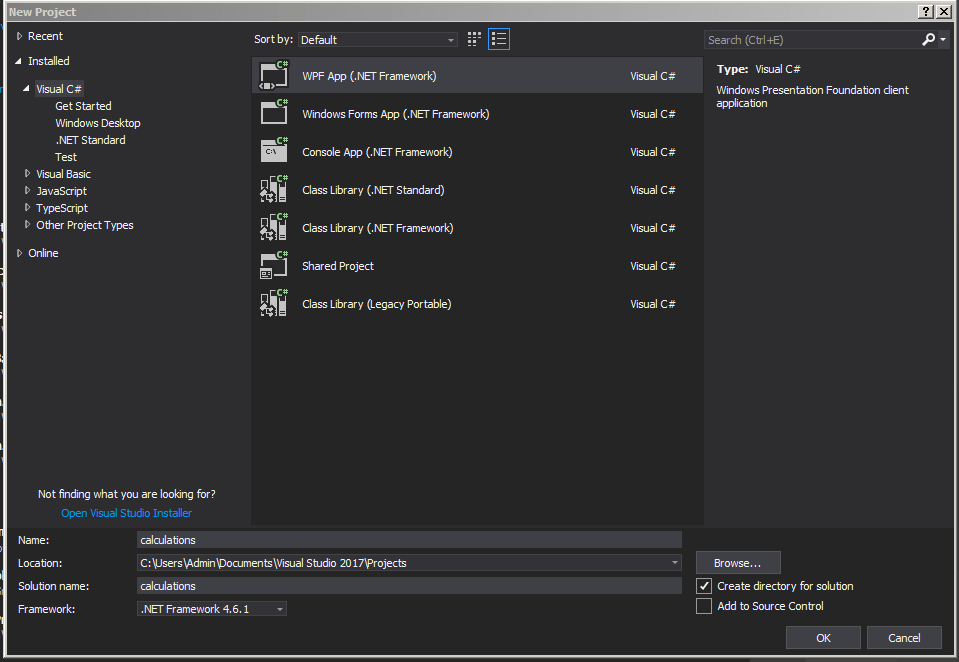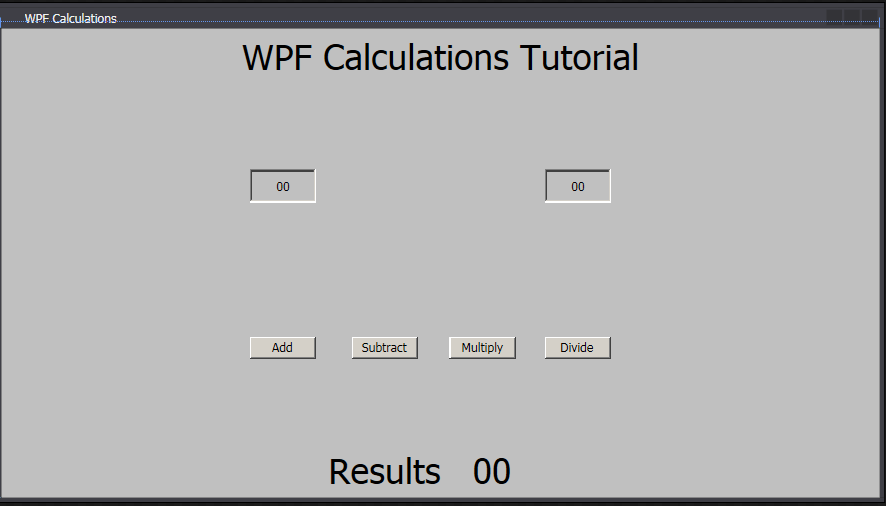# WPF C# Tutorial – Making a Calculator App in Visual Studio

In this tutorial we will create a simple calculator in Visual Studio using WPF C# programming language. The purpose of this tutorial is to get a head start on learning how to make apps in WPF format. We have created several different programs in the classic Windows Form Application template however it’s important for us to learn how to use WPF as its being used more frequently now in app development and games development.

Lesson objectives –

1. Create a full calculator in WPF application using C#
2. Use XAML and C# programming to create the calculator
3. Use Text Boxes and content alignment to style the texts
4. Use dynamic labels and change the values from code directly
5. Add 4 buttons to the calculator to do the adding, subtracting, multiplying and dividing
6. Add 4 events to the buttons
7. Converting strings to double and completing calculations
8. Rounding down decimal numbers and showing on the label

To begin start Visual Studio and click on new projectMake sure you select the WPF App and name the project calculator. Click OK to start.This is the empty app. We will start adding our app elements to the form via XML codes.

```<Window x:Class="calculations.MainWindow"

xmlns="http://schemas.microsoft.com/winfx/2006/xaml/presentation"

xmlns:x="http://schemas.microsoft.com/winfx/2006/xaml"

xmlns:d="http://schemas.microsoft.com/expression/blend/2008"

xmlns:mc="http://schemas.openxmlformats.org/markup-compatibility/2006"

xmlns:local="clr-namespace:calculations"

mc:Ignorable="d"

Title="MainWindow" Height="450" Width="800">

<Grid>

</Grid>

</Window>```

Above is the empty XML codes. We will start adding the components to it but first we need to change the title of the app.

```<Window x:Class="calculations.MainWindow"

xmlns="http://schemas.microsoft.com/winfx/2006/xaml/presentation"

xmlns:x="http://schemas.microsoft.com/winfx/2006/xaml"

xmlns:d="http://schemas.microsoft.com/expression/blend/2008"

xmlns:mc="http://schemas.openxmlformats.org/markup-compatibility/2006"

xmlns:local="clr-namespace:calculations"

mc:Ignorable="d"

Title="WPF Calculations" Height="450" Width="800">

<Grid>

</Grid>

</Window>```

The line Title=”MainWindow” change it to Title=”WPF Calculations” this will change the apps title. The main components are going to be added to the program through the <grid> … </grid> tags. Everything we will need to include will need to go inside that tag.

```<Window x:Class="calculations.MainWindow"

xmlns="http://schemas.microsoft.com/winfx/2006/xaml/presentation"

xmlns:x="http://schemas.microsoft.com/winfx/2006/xaml"

xmlns:d="http://schemas.microsoft.com/expression/blend/2008"

xmlns:mc="http://schemas.openxmlformats.org/markup-compatibility/2006"

xmlns:local="clr-namespace:calculations"

mc:Ignorable="d"

Title="WPF Calculations" Height="450" Width="800">

<Grid>

<Label HorizontalAlignment="Center" FontSize="32">WPF Calculations Tutorial</Label>

<TextBox VerticalContentAlignment="Center" TextAlignment="Center" Name="numberA" Margin="224,127,508,266" Width="60" Height="30">00</TextBox>

<TextBox VerticalContentAlignment="Center" TextAlignment="Center" Name="numberB" Margin="490,127,242,266" Width="60" Height="30">00</TextBox>

</Grid>

</Window>```

Above we are adding few components inside the GRID tags. The first component we added to the code is a LABEL <Label> … </Label> Anything in middle of the Label will be seen as the text. We are also adding some properties to the label.

<Label HorizontalAlignment=”Center” FontSize=”32″>WPF Calculations Tutorial</Label>

Inside the label Tag we first used horizontal alignment to centre of the screen and the font size to 32.

<TextBox VerticalContentAlignment=”Center” TextAlignment=”Center” Name=”numberA” Margin=”224,127,508,266″ Width=”60″ Height=”30″>00</TextBox>

The second tag we are using here is a text box. This one will have a vertical alignment to centre, text alignment to centre this will show the text box contents to centre. We will also add a Name for this text box to numberA, the name property allows us to call the text box from the code. We are also using Margin to 224, 127, 508 and 266 this will position to the box as you see on the screen shot. The text box also has Width of 60 and Height of 30. Once again the contents of the text box will be between the tags <TextBox> 00 </TextBox>.

<TextBox VerticalContentAlignment=”Center” TextAlignment=”Center” Name=”numberB” Margin=”490,127,242,266″ Width=”60″ Height=”30″>00</TextBox>

The third tag is another text box. It has similar values as the numberA text box but this one has the name numberB and margin 490, 127, 242 and 266.

NumberA and NumberB text boxes will be used to hold the two numbers we want to calculate. Next part is adding the buttons.If you want to change the positions later you can also drag the label or text boxes to different positions. For now we can leave it as it is. Let’s start adding the buttons.

```<Window x:Class="calculations.MainWindow"

xmlns="http://schemas.microsoft.com/winfx/2006/xaml/presentation"

xmlns:x="http://schemas.microsoft.com/winfx/2006/xaml"

xmlns:d="http://schemas.microsoft.com/expression/blend/2008"

xmlns:mc="http://schemas.openxmlformats.org/markup-compatibility/2006"

xmlns:local="clr-namespace:calculations"

mc:Ignorable="d"

Title="WPF Calculations" Height="450" Width="800">

<Grid>

<Label HorizontalAlignment="Center" FontSize="32">WPF Calculations Tutorial</Label>

<TextBox VerticalContentAlignment="Center" TextAlignment="Center" Name="numberA" Margin="224,127,508,266" Width="60" Height="30">00</TextBox>

<TextBox VerticalContentAlignment="Center" TextAlignment="Center" Name="numberB" Margin="490,127,242,266" Width="60" Height="30">00</TextBox>

<Button Width="60" Height="20" Margin="316,278,416,125">Subtract</Button>

<Button Width="60" Height="20" Margin="404,278,328,125">Multiply</Button>

<Button Width="60" Height="20" Margin="490,278,242,125">Divide</Button>

</Grid>

</Window>```

Above in the code we are adding 4 different buttons to the app. As you can see all of the buttons have similar settings to them except for the margins. They will all be aligned horizontally across the form. The buttons text will state Add, Subtract, Multiply and Divide. Each button will have an event attached to them and we will add them all shortly.This is the screen shot of all 4 buttons been implemented to the form.

```<Window x:Class="calculations.MainWindow"

xmlns="http://schemas.microsoft.com/winfx/2006/xaml/presentation"

xmlns:x="http://schemas.microsoft.com/winfx/2006/xaml"

xmlns:d="http://schemas.microsoft.com/expression/blend/2008"

xmlns:mc="http://schemas.openxmlformats.org/markup-compatibility/2006"

xmlns:local="clr-namespace:calculations"

mc:Ignorable="d"

Title="WPF Calculations" Height="450" Width="800">

<Grid>

<Label HorizontalAlignment="Center" FontSize="32">WPF Calculations Tutorial</Label>

<TextBox VerticalContentAlignment="Center" TextAlignment="Center" Name="numberA" Margin="224,127,508,266" Width="60" Height="30">00</TextBox>

<TextBox VerticalContentAlignment="Center" TextAlignment="Center" Name="numberB" Margin="490,127,242,266" Width="60" Height="30">00</TextBox>

<Button Width="60" Height="20" Margin="316,278,416,125">Subtract</Button>

<Button Width="60" Height="20" Margin="404,278,328,125">Multiply</Button>

<Button Width="60" Height="20" Margin="490,278,242,125">Divide</Button>

<Label VerticalAlignment="Bottom" FontSize="32" HorizontalAlignment="Center" Margin="290,0,391,0">Results</Label>

<Label Name="result" VerticalAlignment="Bottom" FontSize="32" HorizontalAlignment="Center" Margin="420,0,328,0" Width="140">00</Label>

</Grid>

</Window>```

We need to add two more labels to the form. One will show the text result and the other will be used display the results to the form.

<Label VerticalAlignment=”Bottom” FontSize=”32″ HorizontalAlignment=”Center” Margin=”290,0,391,0″>Results</Label>

This label will be used to show the text results on the screen.

<Label Name=”result” VerticalAlignment=”Bottom” FontSize=”32″ HorizontalAlignment=”Center” Margin=”420,0,328,0″ Width=”140″>00</Label>

Notice that this label has a name attached to it, we will use the name to control the label from the code.This is the final result of the form. All of the elements have been added now we will need to link the buttons to events.

### 2 responses to “WPF C# Tutorial – Making a Calculator App in Visual Studio”

1. Dima says:

How I need to change (NumberA.Text), (NumberB.Text) and whereat?

2. Anhar Ali says:

Hi, Thank you for bringing to my attention, there was a small spelling error which led to the numberA and numberB texboxes not being recognised. I have fixed that now in the source code. Both of them are the text boxes you add to the xaml file and then we call them to capture the input from them to do the calculations.